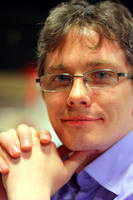Участник:Denis Kochedykov

Перейти к: навигация, поискKochedykov Denis, J.P.Morgan quantitative research, CC RAS (PhD candidate). Scientific advisor: Dr. Konstantin V. Vorontsov. Scientific interests statistical learning theory, generalization ability, combinatorics, statistics.

Pulications

1. Kochedykov D.A. (2011) “A Combinatorial Approach to Hypothesis Similarity in Generalization Bounds” (English+Russian), submitted.
2. Kochedykov D.A. (2010) “Combinatorial shell bounds for generalization ability” (Russian + English), “Pattern Recognition and Image Analysis”, 2010, 4.
3. Kochedykov D.A. (2009) “Connectivity structures in hypotheses’ space and generalization bounds”. Mathematical methods for pattern recognition 2009 (in Russian)
4. Kochedykov D.A (2008) “Combinatorial generalization bounds using the splitting of hypothesis spaces”. Contemporary problems of fundamental and applied sciences 2008 (in Russian)
5. Kochedykov D.A, Vorontsov K.V.(2007) “Information measures for rule induction algorithms”. Contemporary problems of fundamental and applied sciences 2007 (in Russian).
6. Kochedykov D.A, Ivakhnenko A.A, Vorontsov K.V.(2007) “Logical classification algorithms for risk management”. Mathematical methods for pattern recognition 2007 (in Russian).
7. Kochedykov D.A, Vorontsov K.V. (2006) “Meta-learning for rule induction algorithms”. Intelligent information processing 2006 (in Russian).
8. Kochedykov D.A, Ivakhnenko A.A, Vorontsov K.V. (2005) “Credit scoring system based on logical classification algorithms”. Mathematical methods for pattern recognition 2005 (in Russian).